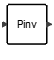# MatrixPseudoInverse

This block outputs the inverse of a non square input matrix using the SVD (Singular Value Decomposition) operation. When the input is an M-by-N matrix, the output is an N-by-M matrix.MatrixOperations

## Description

The MatrixPseudoInverse block outputs the inverse of a non square input matrix using the SVD (Singular Value Decomposition) operation.

The input matrix can be either of type Double or type Complex.

When the input is an M-by-N matrix, the output is an N-by-M matrix.

If the SVD of A is: A=USV' then the pseudoinverse X of A is given by: X=VS"U'

where S"(i,j)=1/S(i,j) (if S(i,j) =0), U' and V are respectively the transpose of U and V'.

and we have A*X*A=A and X*A*X=X. Both A*X and X*A are Hermitian matrices.

A warning message is printed if the input is badly scaled or nearly singular.

## ParametersNameLabelDescriptionData TypeValid Values

tolerance

Tolerance

Tolerance of the computation.

Scalar

externalActivation

External activation

Specifies whether the block receives an external activation or inherits its activation through its regular input ports. When External Activation is selected, an additional activation port is added to the block. By default, external activation is not selected.

Number

0
1

## Ports

NameTypeDescriptionIO TypeNumber

Port 1

explicit

output

1

Port 2

explicit

input

1

Port 3

activation

input

externalActivation

NameValueDescription

always active

no

direct-feedthrough

yes

zero-crossing

no

mode

no

continuous-time state

no

discrete-time state

no